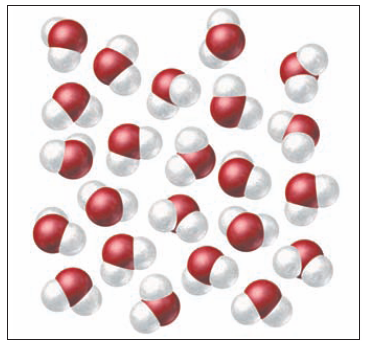×
Get Full Access to Introductory Chemistry - 5 Edition - Chapter 12 - Problem 105p
Get Full Access to Introductory Chemistry - 5 Edition - Chapter 12 - Problem 105p

×

# Solved: Consider the molecular view of water shown here. Pick a molecule in the interiorISBN: 9780321910295 34

## Solution for problem 105P Chapter 12

Introductory Chemistry | 5th Edition

• Textbook Solutions
• 2901 Step-by-step solutions solved by professors and subject experts
• Get 24/7 help from StudySoup virtual teaching assistantsIntroductory Chemistry | 5th Edition

4 5 1 399 Reviews
20
4
Problem 105P

PROBLEM 105P

Consider the molecular view of water shown here. Pick a molecule in the interior and draw a line to each of its direct neighbors. Pick a molecule near the edge (analogous to a molecule on the surface in three dimensions) and do the same. Which molecule has the most neighbors? Which molecule is more likely to evaporate?Step-by-Step Solution:

Solution 105P

Here, we are going draw a line to each of its edge and interior molecule .

Step 1:

The diagram of the edge and interior molecule is as followsStep 2 of 2

##### ISBN: 9780321910295

Unlock Textbook Solution